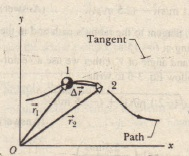# Average Velocity and Instantaneous Velocity

Average Velocity and Instantaneous Velocity

If a particle moves through a displacement t:.r in a time interval , then its average velocity v is,The direction of the instantaneous velocity V of a particle is always tangent to the particle’s path at the particle’s position,

The result is the same in three dimensions: v is always tangent to the particle’s path. To write  in unit vector form, we substitute for r,This equation can be simplified somewhat by writing it asThe displacement  of a particleduring a time interval fromposition 1 with position vector  attime  to position 2 with position vectorat time 12, The tangent to theparticle path at position I is shown.

where the scalar components of are